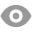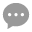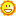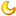# 四周法则交易系统测试和代码汇总196 |8 |王博 | 2020-4-28 11:46:48 | 显示全部楼层 |阅读模式
 四周法则交易系统定义与测试 1）定义四周法则做多系统： 文字描述1：最高价突破20日新高 量化定义：当日最高价=近20日（含当日）最高价 文字描述2：最低价突破20日新低 量化定义：当日最低价=近20日（含当日）最低价 定义测试的买卖逻辑：买入条件：当日最高价突破20日新高，以当日收盘价买入平仓条件：当日最低价突破20日新低，以当日收盘价平仓 2）定义四周法则做空系统： 文字描述1：最低价突破20日新低 量化定义：当日最低价=近20日（含当日）最低价 文字描述2：最高价突破20日新高 量化定义：当日最高价=近20日（含当日）最高价 定义测试的买卖逻辑：买入条件：当日最低价突破20日新低，以当日收盘价买入平仓条件：当日最高价突破20日新高，以当日收盘价平仓（相关阅读：《四周法则交易系统的原理，股票四周法则公式改进策略》）

 测试一：(1) 四周法则做多系统 【测试代码】//定义仓位FUND:=10000000;LOTS:=INTPART(FUND*0.8/(CLOSE+FEE));//计算手数，使每次开仓金额都是800W //定义ATRTR:=MAX(MAX((H-L),ABS(REF(C,1)-H)),ABS(REF(C,1)-L));//求最高价减去最低价，一个周期前的收盘价减去最高价的绝对值，一个周期前的收盘价减去最低价的绝对值，这三个值中的最大值ATR:=MA(TR,26);//求N个周期内的TR的简单移动平均 //定义四周法则SZFZ1:H=HHV(H,20);SZFZ2: L=LLV(L,20); SZFZ1,BK(LOTS);SZFZ2,SP(BKVOL);

 (2) 四周法则做空系统 【测试代码】//定义仓位FUND:=10000000;LOTS:=INTPART(FUND*0.8/(CLOSE+FEE));//计算手数，使每次开仓金额都是800W //定义ATRTR:=MAX(MAX((H-L),ABS(REF(C,1)-H)),ABS(REF(C,1)-L));//求最高价减去最低价，一个周期前的收盘价减去最高价的绝对值，一个周期前的收盘价减去最低价的绝对值，这三个值中的最大值ATR:=MA(TR,26);//求N个周期内的TR的简单移动平均 //定义四周法则SZFZ1:=H=HHV(H,20);SZFZ2:=L=LLV(L,20); SZFZ2,BK(LOTS);SZFZ1,SP(BKVOL);

 测试二：(1) 四周法则做多系统优化突破周期 【测试代码】//定义仓位FUND:=10000000;LOTS:=INTPART(FUND*0.8/(CLOSE+FEE));//计算手数，使每次开仓金额都是800W //定义ATRTR:=MAX(MAX((H-L),ABS(REF(C,1)-H)),ABS(REF(C,1)-L));//求最高价减去最低价，一个周期前的收盘价减去最高价的绝对值，一个周期前的收盘价减去最低价的绝对值，这三个值中的最大值ATR:=MA(TR,26);//求N个周期内的TR的简单移动平均 //定义四周法则SZFZ1:=H=HHV(H,N);SZFZ2:=L=LLV(L,N); SZFZ1,BK(LOTS);SZFZ2,SP(BKVOL);

 (2) 四周法则做空系统优化突破周期 【测试代码】//定义仓位FUND:=10000000;LOTS:=INTPART(FUND*0.8/(CLOSE+FEE));//计算手数，使每次开仓金额都是800W //定义ATRTR:=MAX(MAX((H-L),ABS(REF(C,1)-H)),ABS(REF(C,1)-L));//求最高价减去最低价，一个周期前的收盘价减去最高价的绝对值，一个周期前的收盘价减去最低价的绝对值，这三个值中的最大值ATR:=MA(TR,26);//求N个周期内的TR的简单移动平均 //定义四周法则SZFZ1:=H=HHV(H,N);SZFZ2:=L=LLV(L,N); SZFZ2,BK(LOTS);SZFZ1,SP(BKVOL);

 测试三：(1) 四周法则做多系统+成交量 【测试结果】 //定义仓位 FUND:=10000000; LOTS:=INTPART(FUND*0.8/(CLOSE+FEE));//计算手数，使每次开仓金额都是800W //定义ATR TR:=MAX(MAX((H-L),ABS(REF(C,1)-H)),ABS(REF(C,1)-L));//求最高价减去最低价，一个周期前的收盘价减去最高价的绝对值，一个周期前的收盘价减去最低价的绝对值，这三个值中的最大值 ATR:=MA(TR,26);//求N个周期内的TR的简单移动平均 //定义四周法则 SZFZ1:=H=HHV(H,20);//突破20日新高 SZFZ2:=L=LLV(L,20);//突破20日新低 //定义成交量 CJL:=VOL>MA(VOL,N);//成交量大于N日均量 SZFZ1 AND CJL,BK(LOTS); SZFZ2,SP(BKVOL);

 (2) 四周法则做空系统+成交量 【测试结果】 //定义仓位 FUND:=10000000; LOTS:=INTPART(FUND*0.8/(CLOSE+FEE));//计算手数，使每次开仓金额都是800W //定义ATR TR:=MAX(MAX((H-L),ABS(REF(C,1)-H)),ABS(REF(C,1)-L));//求最高价减去最低价，一个周期前的收盘价减去最高价的绝对值，一个周期前的收盘价减去最低价的绝对值，这三个值中的最大值 ATR:=MA(TR,26);//求N个周期内的TR的简单移动平均 //定义成交量 CJL:=VOL>MA(VOL,N);//成交量大于N日均量 //定义四周法则 SZFZ1:=H=HHV(H,20); SZFZ2:=L=LLV(L,20); SZFZ2 AND CJL,BK(LOTS); SZFZ1,SP(BKVOL);

 测试四：(1) 四周法则做多系统+OBV 【测试结果】 //定义仓位 FUND:=10000000; LOTS:=INTPART(FUND*0.8/(CLOSE+FEE));//计算手数，使每次开仓金额都是800W //定义ATR TR:=MAX(MAX((H-L),ABS(REF(C,1)-H)),ABS(REF(C,1)-L));//求最高价减去最低价，一个周期前的收盘价减去最高价的绝对值，一个周期前的收盘价减去最低价的绝对值，这三个值中的最大值 ATR:=MA(TR,26);//求N个周期内的TR的简单移动平均 //定义OBV OBV:=SUM(IFELSE(CLOSE>REF(CLOSE,1),VOL,IFELSE(CLOSEHV(OBV,N); //定义四周法则 SZFZ1:H>HV(H,20); SZFZ2: L

 (2) 四周法则做空系统+OBV 【测试结果】 //定义仓位 FUND:=10000000; LOTS:=INTPART(FUND*0.8/(CLOSE+FEE));//计算手数，使每次开仓金额都是800W //定义ATR TR:=MAX(MAX((H-L),ABS(REF(C,1)-H)),ABS(REF(C,1)-L));//求最高价减去最低价，一个周期前的收盘价减去最高价的绝对值，一个周期前的收盘价减去最低价的绝对值，这三个值中的最大值 ATR:=MA(TR,26);//求N个周期内的TR的简单移动平均 //定义OBV OBV:=SUM(IFELSE(CLOSE>REF(CLOSE,1),VOL,IFELSE(CLOSE

 您需要登录后才可以回帖 登录 | 立即注册 本版积分规则 回帖后跳转到最后一页87主题 1219积分1219